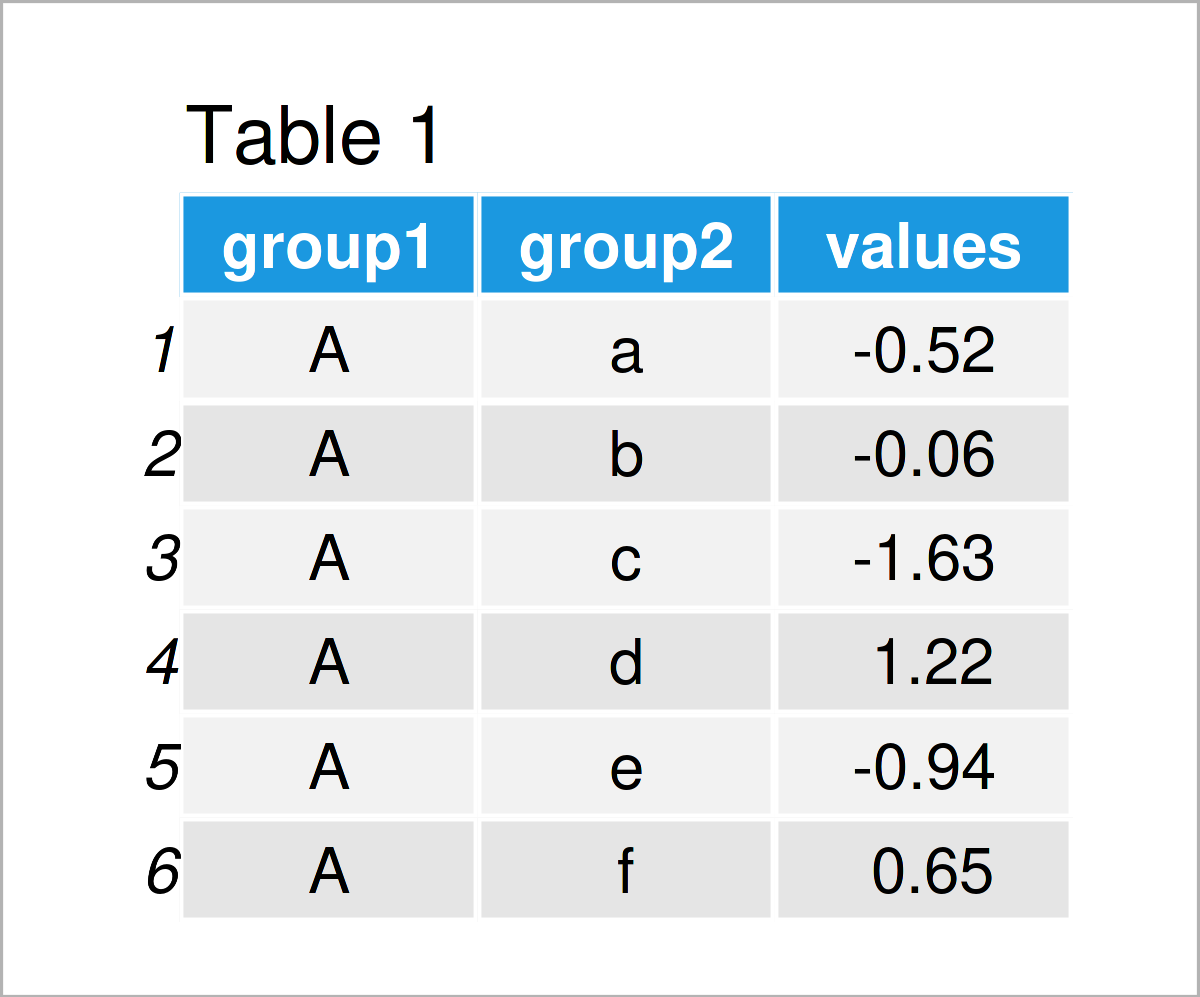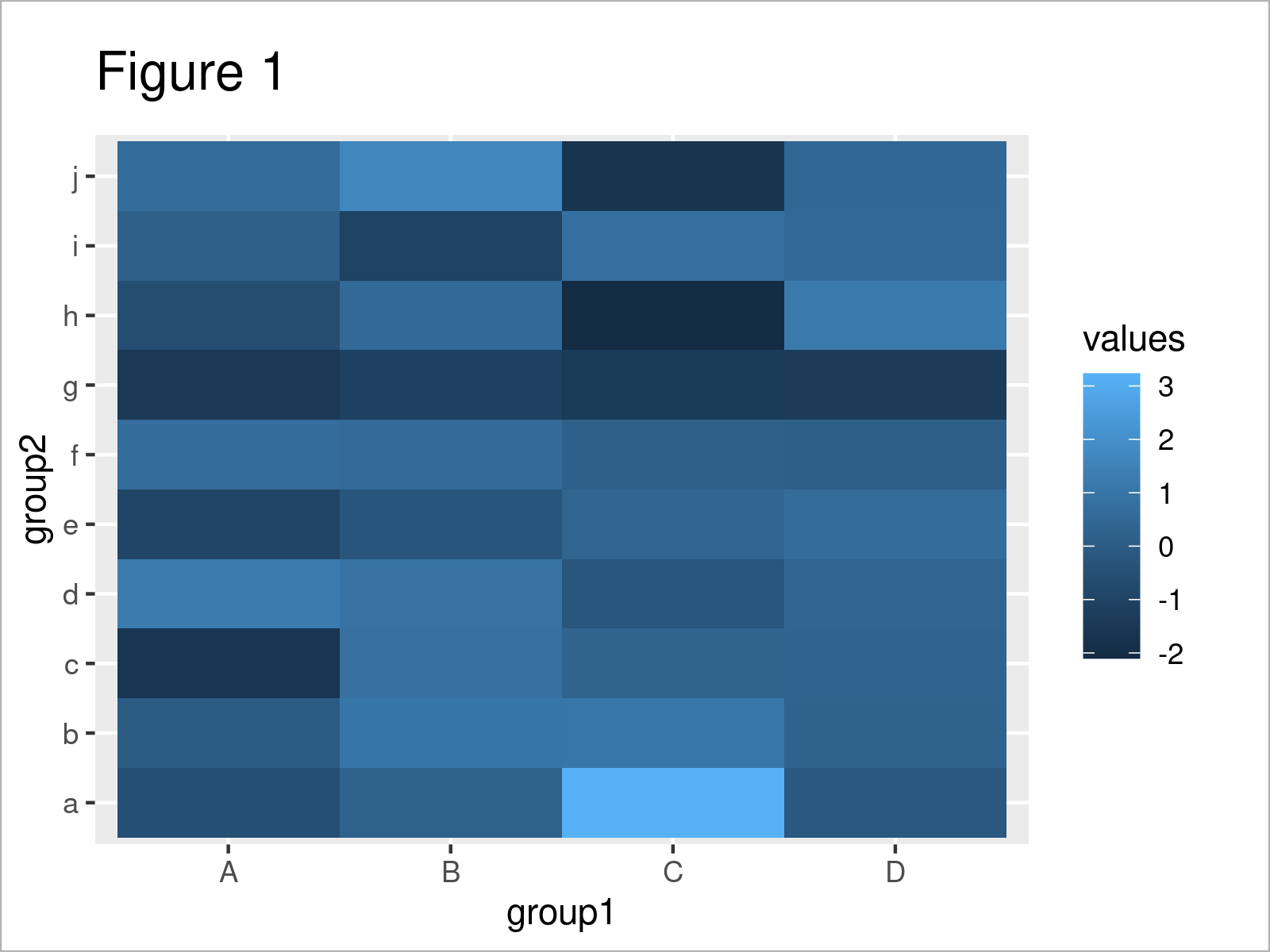# Add Values to Heatmap in R (Example)

In this tutorial, I’ll illustrate how to draw a heatmap with values in the R programming language.

So now the part you have been waiting for – the R code.

## Example Data, Software Packages & Basic Graphic

Consider the following example data:

```set.seed(328576)                              # Create example data
data <- data.frame(group1 = rep(LETTERS[1:4], each = 10),
group2 = letters[1:10],
values = round(rnorm(40), 2))Have a look at the table that has been returned after executing the previously shown R programming syntax. It shows the first six lines of our example data, and that our data is made of the three columns “group1”, “group2”, and “values”. The variables group1 and group2 have the character class and the variable values is numerical.

We also need to install and load the ggplot2 package to RStudio, in order to use the corresponding functions:

```install.packages("ggplot2")                   # Install & load ggplot2
library("ggplot2")```

As a next step, we can draw our data.

```ggp <- ggplot(data, aes(group1, group2)) +    # Create default ggplot2 heatmap
geom_tile(aes(fill = values))
ggp                                           # Draw default ggplot2 heatmap```By running the previous code we have plotted Figure 1, i.e. a heatmap without values.

## Example: Add Values to ggplot2 Heatmap Using geom_text() Function

This example illustrates how to create a heatmap with values using the R programming language.

For this task, we can use the geom_text function as shown below:

```ggp +                                         # Add values to heatmap
geom_text(aes(label = values))```As shown in Figure 2, the previous R syntax has managed to create a heatmap with values.

However, as you can see some of the values cannot be read, since the color of the heatmap cells is similar to the text color.

For that reason, we can modify the heatmap color using the scale_fill_gradient function:

```ggp +                                         # Add values & modify color
geom_text(aes(label = values)) +
scale_fill_gradient(low = "white", high = "#1b98e0")```By executing the previous R syntax we have created Figure 3, i.e. a heatmap with values and modified colors.

## Video & Further Resources

Please accept YouTube cookies to play this video. By accepting you will be accessing content from YouTube, a service provided by an external third party.If you accept this notice, your choice will be saved and the page will refresh.

In addition, you may want to read some of the related articles on this website.

To summarize: In this R post you have learned how to create a heatmap graphic with values. Don’t hesitate to tell me about it in the comments section, if you have any additional comments or questions.

Subscribe to the Statistics Globe Newsletter